# Test: Pulse Modulation

## 10 Questions MCQ Test GATE ECE (Electronics) 2023 Mock Test Series | Test: Pulse Modulation

Description
Attempt Test: Pulse Modulation | 10 questions in 30 minutes | Mock test for GATE preparation | Free important questions MCQ to study GATE ECE (Electronics) 2023 Mock Test Series for GATE Exam | Download free PDF with solutions
QUESTION: 1

### The Nyquist rate for a continuous-time signal (x) is x(t) = 6cos50πt + 20sin300πt - 10cos100πt

Solution:

Given,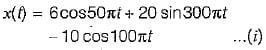Also,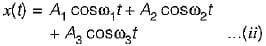Comparing equations (I) and (II), we have: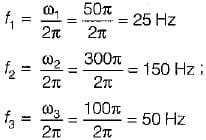Thus, the highest frequency component of the given message signal will be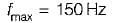∴ Nyquist rate = 2fmax = 2 x 150 = 300 Hz

QUESTION: 2

### Which of the following pulse modulation systems is analog?

Solution:

PAM, PWM and PPM are examples of analog pulse modulation techniques while PCM is a digital pulse modulation.

QUESTION: 3

### The Nyquist interval for the signal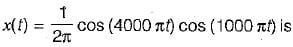Solution:

Given signal is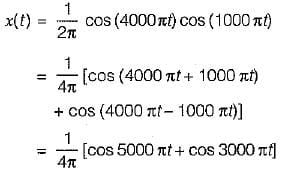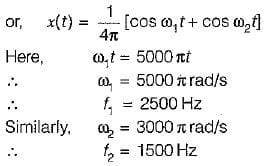Hence, the maximum frequency present in x(t) is f1= 2500 Hz
∴ Nyquist rate is given as
fs = 2fm
(where, fm = Maximum frequency present in the signal)
Here, fm = f1 = 2500 Hz
∴ Nyquist rate is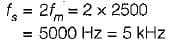Nyquist interval is given as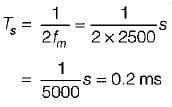QUESTION: 4

A telephone signal with cut-off frequency of 4 kHz is digitized into 8 bit PCM, sampled at Nyquist rate. The quantization S/N ratio is

Solution: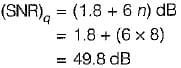QUESTION: 5

A sine wave of frequency fm and amplitude Am is applied to a delta modulator having step size Δ. The slope overload distortion will not occur if

Solution:

Let, x(t) = Am sin (2πfmt)
The slope of x(t) will be maximum when derivative of x(t) w.r.t. 't' will be maximum.
Maximum slope = Δ/Ts
Hence, for slope overload distortion not to occur,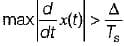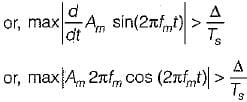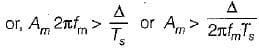Hence, slope overload distortion doesn’t occur if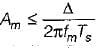QUESTION: 6

The output signal to noise ratio (SNR) of a 10-bit PCM was found to be 30 dB. The desired SNR is 42 dB. It was decided to increase the SNR to the desired value by increasing the number of quantization levels. The percentage increase in the transmission bandwidth required for this SNR is

Solution:

Given, SNR = 30 dB, n = 10,
Desired value of SNR = 42 dB
With the increase in n by 1 bit, the value of SNR increases by 6 dB. Therefore, to increase the value of SNR by 12 dB, it is necessary to increase n by 2,
∴ n = 10 + 2 = 12
Now, BW of PCM system = 1/2 nfs
Therefore, BW with n = 10 is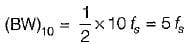and BW for n = 12 is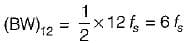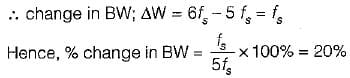QUESTION: 7

The biggest disadvantages of PCM is

Solution:
QUESTION: 8

The bandwidth of TV video plus audio signal is 4.5 MHz. If this signal is converted into PCM bit stream with 1024 quantization levels and the signal is sampled at the rate 20% above Nyquist rate, then the number of bits/sec of the resulting signal is

Solution:

Given,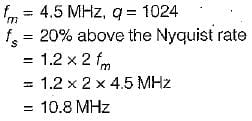Now, quantization level, q = 2n
(n = No. of bits/sec)
∴ 2n = 1024 = 210
or, n = 10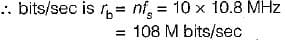QUESTION: 9

Assertion (A) : PCM has much better noise immunity as compared to PAM, PWM and PPM systems.
Reason (R) : PCM does not contain any information in the width or the position of the pulses.

Solution:

In practice, the transmitted pulses usually have slightly sloping sides (edges). As the noise is superimposed on them, the width and the position of the regenerated pulses is changed. This distorts the information contents in the PWM and PPM signals. Since PCM does not contain any information in the width or the position of the pulses, therefore PCM has much better noise immunity as compared to PAM, PWM and PPM systems. Hence, both assertion and reason are true and reason is the correct explanation of assertion.

QUESTION: 10

Assertion (A) : The signaling rate and transmission channel bandwidth is quite large for delta modulation compared to PCM.
Reason (R) : There is no analog to digital converter required in delta modulation unlike PCM.

Solution:

Since, the delta modulation transmits only one bit for one sample, therefore the signaling rate and transmission channel bandwidth is quite small for delta modulation compared to PCM, Hence, assertion is a false statement.
The transmission and receiver implementation is very simple for delta modulation since there is no A/D converter required in delta modulation unlike PCM. Hence, reason is a true statement.Use Code STAYHOME200 and get INR 200 additional OFF Use Coupon Code